PROC MIANALYZE Statement
PROC MIANALYZE <options> ;

Table 57.1 summarizes the options in the PROC MIANALYZE statement.

Table 57.1 Summary of PROC MIANALYZE Options

Option

Description

Input Data Sets

Specifies the COV, CORR, or EST type data set

Specifies the data set for parameter estimates and standard errors

Specifies the data set for parameter estimates

Specifies the data set for parameter information

Specifies the data set for covariance matrices

Specifies the data set formatrices

Statistical Analysis

Specifies parameters under the null hypothesis

Specifies the level for the confidence interval

Specifies the complete-data degrees of freedom

Printed Output

Displays the within-imputation covariance matrix

Displays the between-imputation covariance matrix

Displays the total covariance matrix

Displays multivariate inferences

The following options can be used in the PROC MIANALYZE statement. They are listed in alphabetical order.

ALPHA=specifies that confidence limits are to be constructed for the parameter estimates with confidence level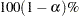, where
. The default is ALPHA=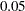.

BCOV

displays the between-imputation covariance matrix.

COVB <(EFFECTVAR=STACKING  |  ROWCOL)> =SAS-data-set

names an input SAS data set that contains covariance matrices of the parameter estimates from imputed data sets. If you provide a COVB= data set, you must also provide a PARMS= data set.

The EFFECTVAR= option identifies the variables for parameters displayed in the covariance matrix and is used only when the PARMINFO= option is not specified. The default is EFFECTVAR= STACKING.

See the section Input Data Sets for a detailed description of the COVB= option.

DATA=SAS-data-set

names an input SAS data set.

If the input DATA= data set is not a specially structured SAS data set, the data set contains both the parameter estimates and associated standard errors. The parameter estimates are specified in the MODELEFFECTS statement and the standard errors are specified in the STDERR statement.

If the data set is a specially structured input SAS data set, it must have a TYPE of EST, COV, or CORR that contains estimates from imputed data sets:

• If TYPE=EST, the data set contains the parameter estimates and associated covariance matrices.

• If TYPE=COV, the data set contains the sample means, sample sizes, and covariance matrices. Each covariance matrix for variables is divided by the sample sizeto create the covariance matrix for parameter estimates.

• If TYPE=CORR, the data set contains the sample means, sample sizes, standard errors, and correlation matrices. The covariance matrices are computed from the correlation matrices and associated standard errors. Each covariance matrix for variables is divided by the sample sizeto create the covariance matrix for parameter estimates.

If you do not specify an input data set with the DATA= or PARMS= option, then the most recently created SAS data set is used as an input DATA= data set. See the section Input Data Sets for a detailed description of the input data sets.

EDF=number

specifies the complete-data degrees of freedom for the parameter estimates. This is used to compute an adjusted degrees of freedom for each parameter estimate. By default, EDF=and the degrees of freedom for each parameter estimate are not adjusted.

MULT
MULTIVARIATE

requests multivariate inference for the parameters. It is based on Wald tests and is a generalization of the univariate inference. See the section Multivariate Inferences for a detailed description of the multivariate inference.

PARMINFO=SAS-data-set

names an input SAS data set that contains parameter information associated with variables PRM1, PRM2,..., and so on. These variables are used as variables for parameters in a COVB= data set. See the section Input Data Sets for a detailed description of the PARMINFO= option.

PARMS <(CLASSVAR= ctype)> =SAS-data-set

names an input SAS data set that contains parameter estimates computed from imputed data sets. When a COVB= data set is not specified, the input PARMS= data set also contains standard errors associated with these parameter estimates. If multivariate inference is requested, you must also provide a COVB= or XPXI= data set.

When the effects contain classification variables, the option CLASSVAR= ctype can be used to identify the associated classification variables when reading the classification levels from observations. The available types are FULL, LEVEL, and CLASSVAL. The default is CLASSVAR= FULL. See the section Input Data Sets for a detailed description of the PARMS= option.

TCOV

displays the total covariance matrix derived by assuming that the population between-imputation and within-imputation covariance matrices are proportional to each other.

THETA0=numbers
MU0=numbers

specifies the parameter values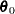under the null hypothesis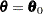in the t tests for location for the effects. If only one numberis specified, that number is used for all effects. If more than one number is specified, the specified numbers correspond to effects in the MODELEFFECTS statement in the order in which they appear in the statement. When an effect contains classification variables, the corresponding value is not used and the test is not performed.

WCOV

displays the within-imputation covariance matrices.

XPXI=SAS-data-set

names an input SAS data set that contains thematrices associated with the parameter estimates computed from imputed data sets. If you provide an XPXI= data set, you must also provide a PARMS= data set. In this case, PROC MIANALYZE reads the standard errors of the estimates from the PARMS= data. The standard errors andmatrices are used to derive the covariance matrices.